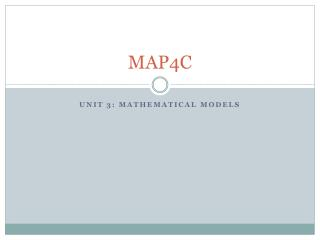# MAP4C - PowerPoint PPT PresentationDownload PresentationMAP4C

MAP4C
Download Presentation## MAP4C

- - - - - - - - - - - - - - - - - - - - - - - - - - - E N D - - - - - - - - - - - - - - - - - - - - - - - - - - -
##### Presentation Transcript

1. MAP4C Unit 3: Mathematical Models

2. One Variable Data A variable is an attribute that can be measured. One-variable data sets give measures of one attribute. One-variable data can be analyzed using mean, median and mode. You can recognize this when you see: Tally charts Frequency Tables Bar Graphs Histograms Pictographs Circle graphs

3. Two Variable Data Two-variable data sets give measures of two attributes for each item in a sample. You can recognize 2-variable data when you see: Ordered pairs Scatter plots Two column tables of values

4. Recognizing Data Types State whether each situation involves one or two-variable data. Justify your choice. Noah researches annual hours of sunshine in Canadian cities. A study compares the length of time children spend playing video games and the time they spend reading. a) As this data can be analyzed with mean, median or mode: this is 1-variable data. b) Two pieces of information are studied and can be plotted on a scatter plot graph. This is 2-variable data.

5. Deciding which type of graph to draw Dylan conducted a survey about students part-time jobs:

6. Deciding which type of graph to draw What graph type would be best to show how many hours each student worked on the weekend? Is this 1 or 2 variable data? Justify. What type of graph would best show a relationship between weekday and weekend hours worked? Is this 1 or 2 variable data? Justify. Graph each situation indicated. Would you expect any correlation in the graph from part b?